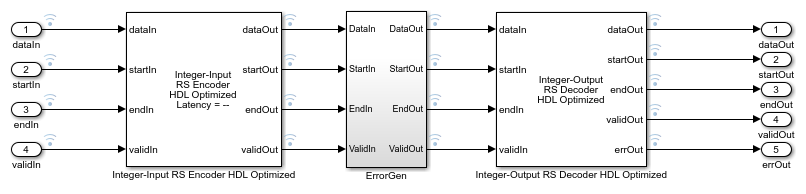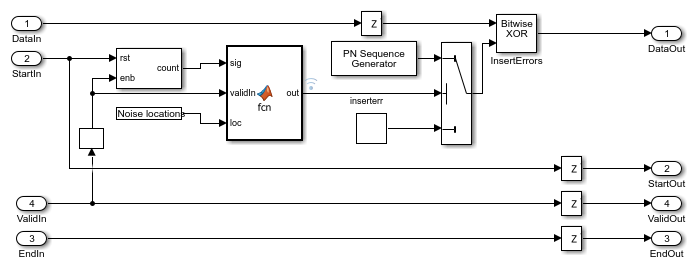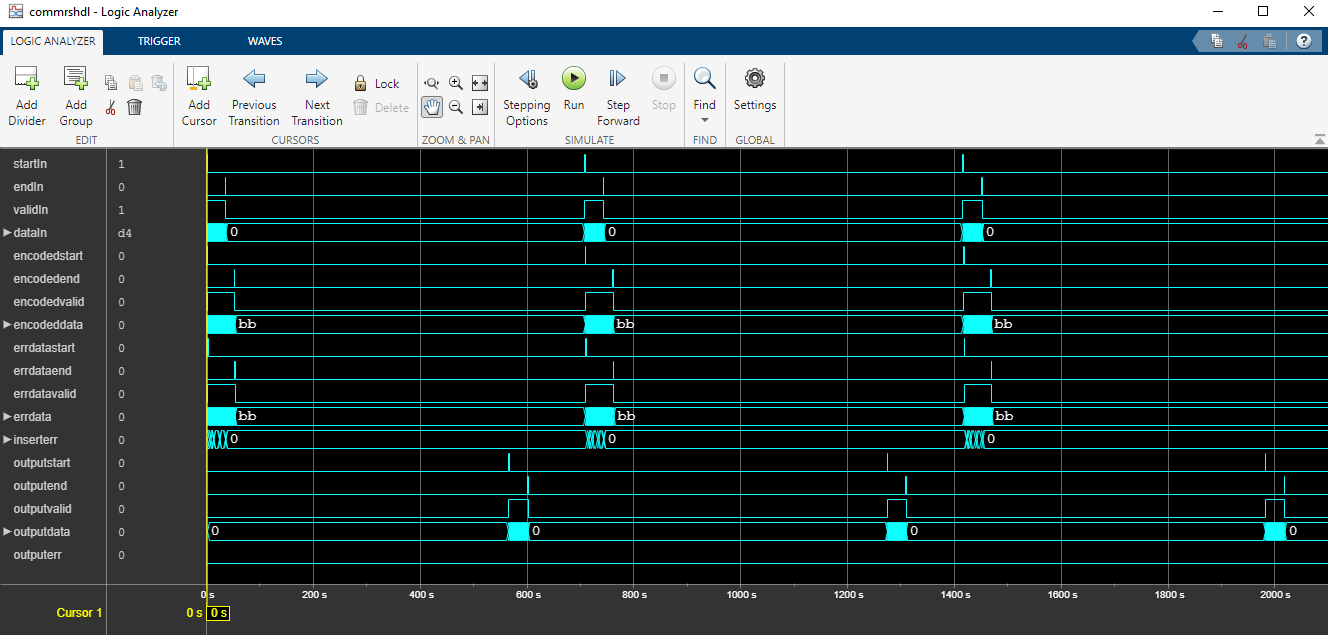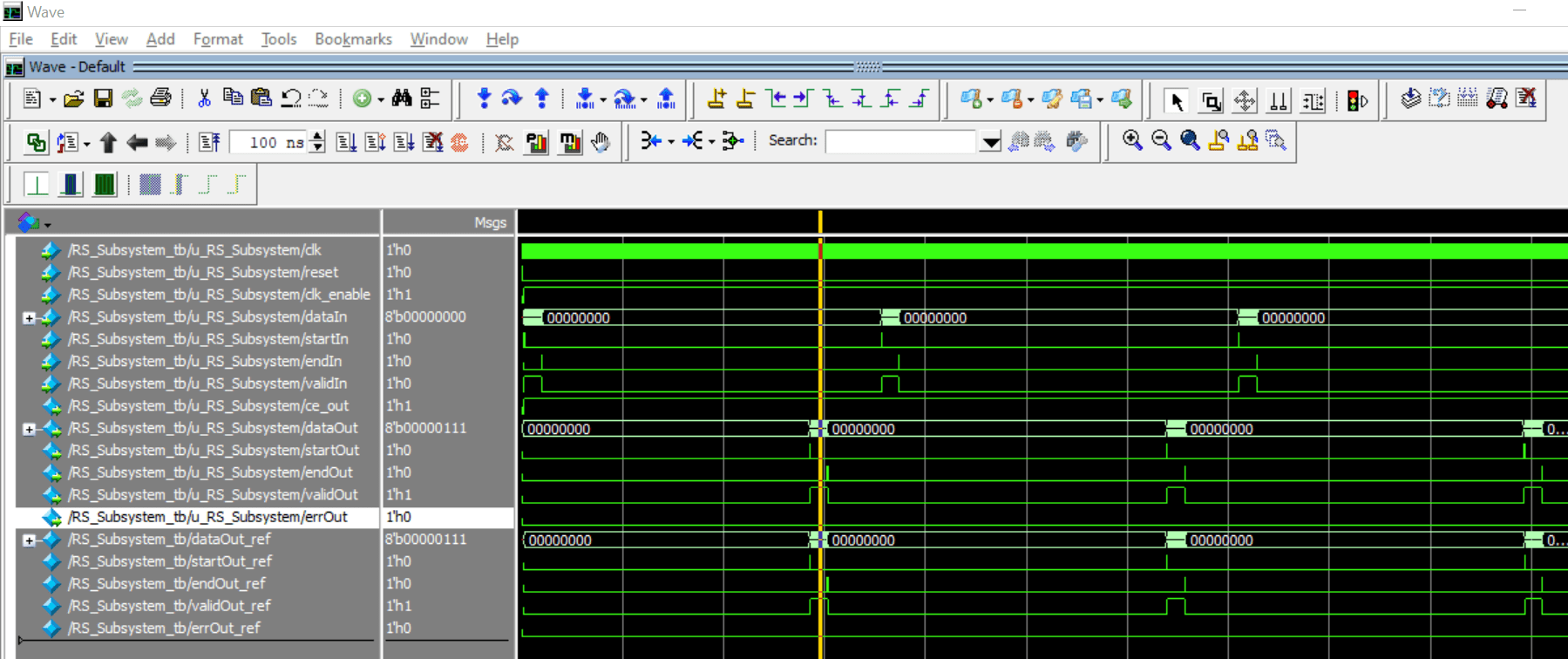# Using HDL Optimized RS Encoder/Decoder Library Blocks

This example shows how to implement encoder and decoder for the IEEE® 802.16 standard [ 1 ] using the HDL Optimized Reed-Solomon (RS) Encoder and Decoder library blocks.

### Introduction

The RS code is a nonbinary block code. A RS code that mapsinformation symbols into a codeword of symbol lengthis denoted as RS(,) code. The symbols for the code are integers betweenand, which represent elements of the finite field GF(). The IEEE 802.16 Broadband Wireless Access standard [ 1 ] employs a Shortening, Puncturing, and Erasures of the RS(255,239) code generated on GF(256), i.e.,,, and. RS encoder introducesparity symbols, which are used by the RS decoder to detect and correct symbol errors. The code can correct up tosymbol errors in each codeword.

This model shows how to use HDL Optimized RS Encoder and Decoder library blocks for simulation and HDL Code generation. It implements the encoding and error correction for the IEEE 802.16 standard. For details about HDL support for HDL Optimized RS Encoder and Decoder blocks, refer to Integer-Input RS Encoder HDL Optimized or Integer-Output RS Decoder HDL Optimized. To learn more about the algorithm used in the blocks refer to [ 2 ].

To open this example model, run the following commands:

modelname = 'commrshdl'; open_system(modelname);### Source

The Source subsystem generates the information symbols for the RS Encoder. To open the Source subsystem, run the following commands:

systemname = [modelname '/Source']; open_system(systemname);One of the messages (information symbols) employed by the IEEE 802.16 standard contains the following 36 bytes (Randomized data specified on page 827 of [ 1 ]).

 message = [D4 BA A1 12 F2 74 96 30 27 D4 88 9C 96 E3 A9 52 B3 15 AB FD 92 53 07 32 C0 62 48 F0 19 22 E0 91 62 1A C1 00].

The Source repeatedly transmits the message followed by a guard interval. The model has parameters messagelength, for the number of symbols in the message to encode; and period, which includes the messagelength and the length of the guard interval. The guard interval between messages accommodates the latency of the encoder adding parity check symbols to the message, and the decoder performing a Chien search. In the initFcn callback of the model, the messagelength is set to 36 and period is set to 236 (which suggest that the guard interval has a length of 200 symbols).

Note that the values of messagelength and period can be varied as desired.

The top-level RS Subsystem contains the HDL Optimized RS Encoder and Decoder blocks. To open the RS subsystem, run the following commands:

systemname = [modelname '/RS Subsystem']; open_system(systemname);The values ofandare set in the InitFcn callback of the model and are used to configure the HDL Optimized RS Encoder and Decoder blocks. The values ofandcannot be changed in this model.

The RS encoder infers a shortened code if the message length is less thansymbols. In this case, it will pad the input message withzeros, encodes the padded message, and appends 16 parity check symbols. The block then removes the added zeros symbols, creating asymbol output.

The field generator polynomial employed by IEEE 802.16 standard is. Accordingly, for both RS encoder and decoder, the Source of primitive polynomial is set as Property, the Primitive polynomial is set as [1 0 0 0 1 1 1 0 1], the Source of B which is the starting power for roots of the primitive polynomial is set as Property, and the B value is set as 0. The code generator polynomial used by IEEE 802.16 standard is, where.

Restrictions onand the codeword lengthare detailed on the Integer-Input RS Encoder block reference page. The ErrorGen subsystem adds noise to the RS encoded message. To open the ErrorGen subsystem, run the following commands:

systemname = [modelname '/RS Subsystem/ErrorGen']; open_system(systemname);The ErrorGen subsystem implements the logic to add noise to the codewords at locations specified in the Noise Locations constant. The location can be changed as desired. In this example, the noise will be added to the 5th, 23rd, 34th, and 12th codewords, corresponding to the symbols F2, 07, 1A, and 9C. The MATLAB® function block outputs logical true only at these four time instances for each packet, and activates a bitwise XOR operation between the original symbols and the noise.

### Output Signals

Run the model using the following command:

sim(modelname); 

### Viewing the Signals

The Logic Analyzer can be used to view multiple signals in one window and viewing signals this way makes it easier to observe transitions. Signals in this model at various stages, namely, before encoding, after encoding, after adding noise, and after decoding are streamed. The blue icon in the model indicates streamed signals. Launch the Logic Analyzer from the model's toolbar.### Analysis of Results

In the Logic Analyzer output the inputdata signal represents the input of the RS encoder block and this is the 36 byte message given in the IEEE 802.16 specification. The encoded data shows the output of the RS encoder block. Note that the IEEE 802.16 specification performs puncturing of the parity bytes and retains only the first four bytes of the 16 bytes. In this demo all 16 bytes of parity are used and the first four bytes of parity are 49, 31, 40, and BF, matching the IEEE 802.16 specification.

The errdata signal represents the encoded data with noise added in the specified noise locations. These noise locations are marked with 1s in the inserterr signal.

The decoded and corrected message out of the RS decoder block is shown by the outputdata signal. Note that the RS decoder block introduces about 3 period lengths of latency. Observe outputdata to see that the errors induced by noise are corrected.

### Generate HDL Code and Test Bench

To check and generate HDL code for this example, you must have an HDL Coder™ license.

Get a unique temporary directory name for the generated files,

 workingdir = tempname;

To check whether there are any issues with the model for HDL code generation, you can run the following command:

 checkhdl('commrshdl/RS Subsystem','TargetDirectory',workingdir);

Enter the following command to generate HDL code:

 makehdl('commrshdl/RS Subsystem','TargetDirectory',workingdir);

Enter the following command to generate the test bench:

 makehdltb('commrshdl/RS Subsystem','TargetDirectory',workingdir);

### ModelSim® Output

The following figure shows the ModelSim HDL simulator after running the generated .do file scripts for the test bench. Compare the ModelSim result with the Simulink® result as plotted before.### Selected References

1. IEEE 802.16: IEEE Standard for Air Interface for Broadband Wireless Access Systems(Revision of IEEE Std 802.16-2009). IEEE-SA. 8 June 2012.

2. George C. Clark Jr, J. Bibb Cain, Error-Correction Coding for Digital Communications, New York: Springer, 1981.